# Visualizing Neural Networks with the Grand Tour

March 16, 2020

### DOI

10.23915/distill.00025

The Grand Tour is a classic visualization technique for high-dimensional point clouds that projects a high-dimensional dataset into two dimensions. Over time, the Grand Tour smoothly animates its projection so that every possible view of the dataset is (eventually) presented to the viewer. Unlike modern nonlinear projection methods such as t-SNE and UMAP, the Grand Tour is fundamentally a linear method. In this article, we show how to leverage the linearity of the Grand Tour to enable a number of capabilities that are uniquely useful to visualize the behavior of neural networks. Concretely, we present three use cases of interest: visualizing the training process as the network weights change, visualizing the layer-to-layer behavior as the data goes through the network and visualizing both how adversarial examples are crafted and how they fool a neural network.

## Introduction

Deep neural networks often achieve best-in-class performance in supervised learning contests such as the ImageNet Large Scale Visual Recognition Challenge (ILSVRC). Unfortunately, their decision process is notoriously hard to interpret, and their training process is often hard to debug. In this article, we present a method to visualize the responses of a neural network which leverages properties of deep neural networks and properties of the Grand Tour. Notably, our method enables us to more directly reason about the relationship between changes in the data and changes in the resulting visualization. As we will show, this data-visual correspondence is central to the method we present, especially when compared to other non-linear projection methods like UMAP and t-SNE.

To understand a neural network, we often try to observe its action on input examples (both real and synthesized). These kinds of visualizations are useful to elucidate the activation patterns of a neural network for a single example, but they might offer less insight about the relationship between different examples, different states of the network as it’s being trained, or how the data in the example flows through the different layers of a single network. Therefore, we instead aim to enable visualizations of the context around our objects of interest: what is the difference between the present training epoch and the next one? How does the classification of a network converge (or diverge) as the image is fed through the network? Linear methods are attractive because they are particularly easy to reason about. The Grand Tour works by generating a random, smoothly changing rotation of the dataset, and then projecting the data to the two-dimensional screen: both are linear processes. Although deep neural networks are clearly not linear processes, they often confine their nonlinearity to a small set of operations, enabling us to still reason about their behavior. Our proposed method better preserves context by providing more consistency: it should be possible to know how the visualization would change, if the data had been different in a particular way.

## Working Examples

To illustrate the technique we will present, we trained deep neural network models (DNNs) with 3 common image classification datasets: MNIST MNIST contains grayscale images of 10 handwritten digits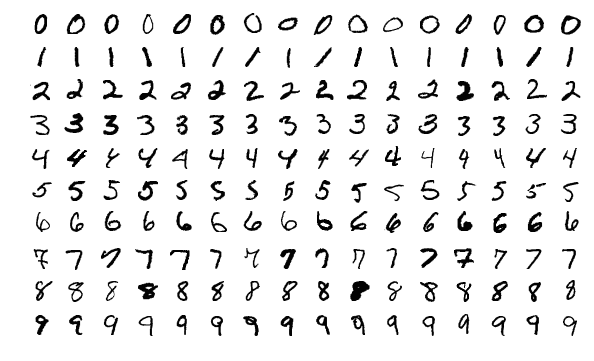Image credit to https://en.wikipedia.org/wiki/File:MnistExamples.png , fashion-MNIST Fashion-MNIST contains grayscale images of 10 types of fashion items: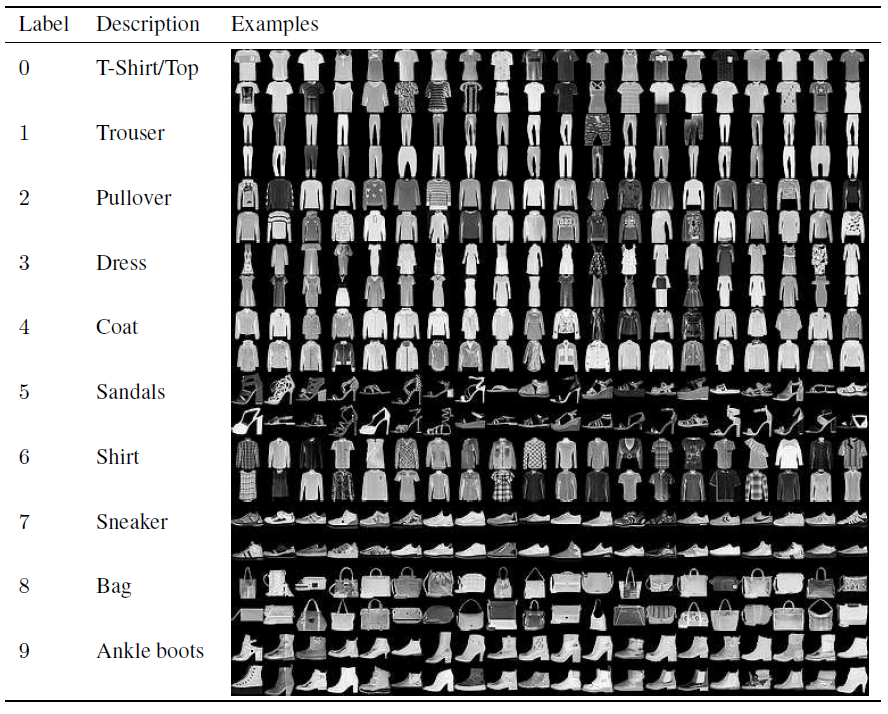Image credit to https://towardsdatascience.com/multi-label-classification-and-class-activation-map-on-fashion-mnist-1454f09f5925 and CIFAR-10 CIFAR-10 contains RGB images of 10 classes of objects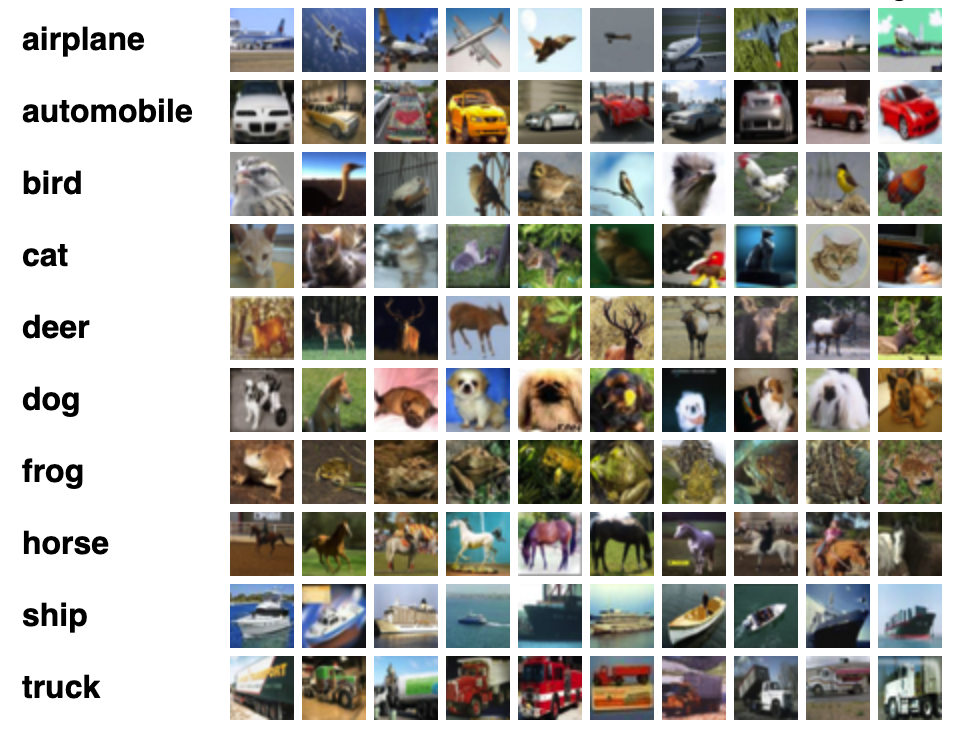Image credit to https://www.cs.toronto.edu/~kriz/cifar.html . While our architecture is simpler and smaller than current DNNs, it’s still indicative of modern networks, and is complex enough to demonstrate both our proposed techniques and shortcomings of typical approaches.

The following figure presents a simple functional diagram of the neural network we will use throughout the article. The neural network is a sequence of linear (both convolutional A convolution calculates weighted sums of regions in the input. In neural networks, the learnable weights in convolutional layers are referred to as the kernel. For exampleImage credit to https://towardsdatascience.com/gentle-dive-into-math-behind-convolutional-neural-networks-79a07dd44cf9.
and fully-connected A fully-connected layer computes output neurons as weighted sum of input neurons. In matrix form, it is a matrix that linearly transforms the input vector into the output vector. ), max-pooling, and ReLU First introduced by Nair and Hinton, ReLU calculates $f(x)=max(0,x)$ for each entry in a vector input. Graphically, it is a hinge at the origin: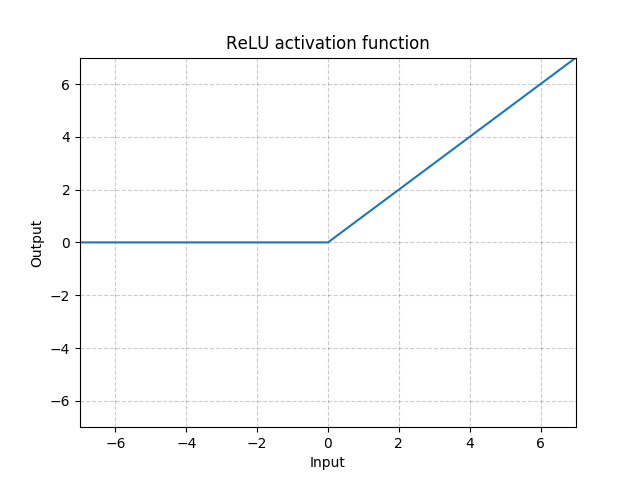Image credit to https://pytorch.org/docs/stable/nn.html#relu layers, culminating in a softmax Softmax function calculates $S(y_i)=\frac{e^{y_i}}{\Sigma_{j=1}^{N} e^{y_j}}$ for each entry ($y_i$) in a vector input ($y$). For example,Image credit to https://ljvmiranda921.github.io/notebook/2017/08/13/softmax-and-the-negative-log-likelihood/ layer.

Even though neural networks are capable of incredible feats of classification, deep down, they really are just pipelines of relatively simple functions. For images, the input is a 2D array of scalar values for gray scale images or RGB triples for colored images. When needed, one can always flatten the 2D array into an equivalent ($w \cdot h \cdot c$) -dimensional vector. Similarly, the intermediate values after any one of the functions in composition, or activations of neurons after a layer, can also be seen as vectors in $\mathbb{R}^n$, where $n$ is the number of neurons in the layer. The softmax, for example, can be seen as a 10-vector whose values are positive real numbers that sum up to 1. This vector view of data in neural network not only allows us represent complex data in a mathematically compact form, but also hints us on how to visualize them in a better way.

Most of the simple functions fall into two categories: they are either linear transformations of their inputs (like fully-connected layers or convolutional layers), or relatively simple non-linear functions that work component-wise (like sigmoid activations Sigmoid calculates $S(x)=\frac{e^{x}}{e^{x}+1}$ for each entry ($x$) in a vector input. Graphically, it is an S-shaped curve.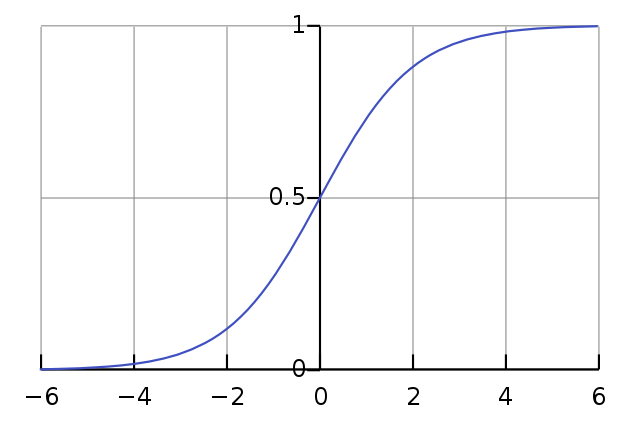Image credit to https://en.wikipedia.org/wiki/Sigmoid_function or ReLU activations). Some operations, notably max-pooling Max-pooling calculates maximum of a region in the input. For example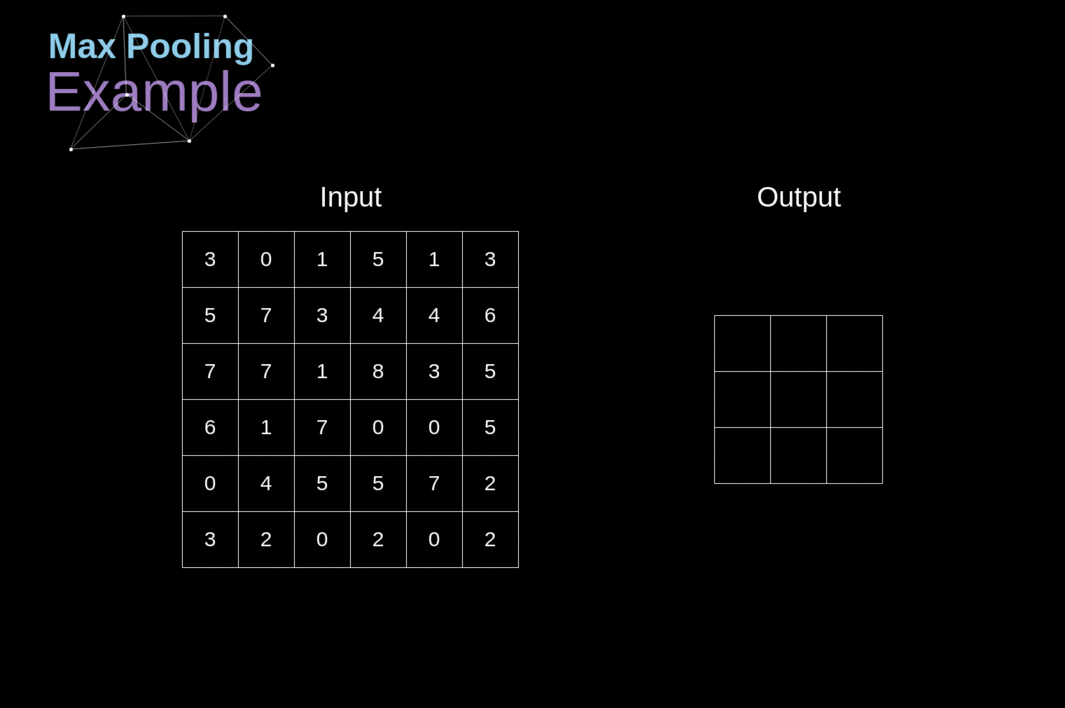Image credit to https://towardsdatascience.com/gentle-dive-into-math-behind-convolutional-neural-networks-79a07dd44cf9 and softmax, do not fall into either categories. We will come back to this later.

The above figure helps us look at a single image at a time; however, it does not provide much context to understand the relationship between layers, between different examples, or between different class labels. For that, researchers often turn to more sophisticated visualizations.

## Using Visualization to Understand DNNs

Let’s start by considering the problem of visualizing the training process of a DNN. When training neural networks, we optimize parameters in the function to minimize a scalar-valued loss function, typically through some form of gradient descent. We want the loss to keep decreasing, so we monitor the whole history of training and testing losses over rounds of training (or “epochs”), to make sure that the loss decreases over time. The following figure shows a line plot of the training loss for the MNIST classifier.

Although its general trend meets our expectation as the loss steadily decreases, we see something strange around epochs 14 and 21: the curve goes almost flat before starting to drop again. What happened? What caused that?

If we separate input examples by their true labels/classes and plot the per-class loss like above, we see that the two drops were caused by the classses 1 and 7; the model learns different classes at very different times in the training process. Although the network learns to recognize digits 0, 2, 3, 4, 5, 6, 8 and 9 early on, it is not until epoch 14 that it starts successfully recognizing digit 1, or until epoch 21 that it recognizes digit 7. If we knew ahead of time to be looking for class-specific error rates, then this chart works well. But what if we didn’t really know what to look for?

In that case, we could consider visualizations of neuron activations (e.g. in the last softmax layer) for all examples at once, looking to find patterns like class-specific behavior, and other patterns besides. Should there be only two neurons in that layer, a simple two-dimensional scatter plot would work. However, the points in the softmax layer for our example datasets are 10 dimensional (and in larger-scale classification problems this number can be much larger). We need to either show two dimensions at a time (which does not scale well as the number of possible charts grows quadratically), or we can use dimensionality reduction to map the data into a two dimensional space and show them in a single plot.

### The State-of-the-art Dimensionality Reduction is Non-linear

Modern dimensionality reduction techniques such as t-SNE and UMAP are capable of impressive feats of summarization, providing two-dimensional images where similar points tend to be clustered together very effectively. However, these methods are not particularly good to understand the behavior of neuron activations at a fine scale. Consider the aforementioned intriguing feature about the different learning rate that the MNIST classifier has on digit 1 and 7: the network did not learn to recognize digit 1 until epoch 14, digit 7 until epoch 21. We compute t-SNE, Dynamic t-SNE, and UMAP projections of the epochs where the phenomenon we described happens. Consider now the task of identifying this class-specific behavior during training. As a reminder, in this case, the strange behavior happens with digits 1 and 7, around epochs 14 and 21 respectively. While the behavior is not particularly subtle&emdash;digit goes from misclassified to correctly classified&emdash; it is quite hard to notice it in any of the plots below. Only on careful inspection we can notice that (for example) in the UMAP plot, the digit 1 which clustered in the bottom in epoch 13 becomes a new tentacle-like feature in epoch 14.

One reason that non-linear embeddings fail in elucidating this phenomenon is that, for the particular change in the data, the fail the principle of data-visual correspondence . More concretely, the principle states that specific visualization tasks should be modeled as functions that change the data; the visualization sends this change from data to visuals, and we can study the extent to which the visualization changes are easily perceptible. Ideally, we want the changes in data and visualization to match in magnitude: a barely noticeable change in visualization should be due to the smallest possible change in data, and a salient change in visualization should reflect a significant one in data. Here, a significant change happened in only a subset of data (e.g. all points of digit 1 from epoch 13 to 14), but all points in the visualization move dramatically. For both UMAP and t-SNE, the position of each single point depends non-trivially on the whole data distribution in such embedding algorithms. This property is not ideal for visualization because it fails the data-visual correspondence, making it hard to infer the underlying change in data from the change in the visualization.

Non-linear embeddings that have non-convex objectives also tend to be sensitive to initial conditions. For example, in MNIST, although the neural network starts to stabilize on epoch 30, t-SNE and UMAP still generate quite different projections between epochs 30, 31 and 32 (in fact, all the way to 99). Temporal regularization techniques (such as Dynamic t-SNE) mitigate these consistency issues, but still suffer from other interpretability issues.

Now, let’s consider another task, that of identifying classes which the neural network tends to confuse. For this example, we will use the Fashion-MNIST dataset and classifier, and consider the confusion among sandals, sneakers and ankle boots. If we know ahead of time that these three classes are likely to confuse the classifier, then we can directly design an appropriate linear projection, as can be seen in the last row of the following figure (we found this particular projection using both the Grand Tour and the direct manipulation technique we later describe). The pattern in this case is quite salient, forming a triangle. T-SNE, in contrast, incorrectly separates the class clusters (possibly because of an inappropriately-chosen hyperparameter). UMAP successfully isolates the three classes, but even in this case it’s not possible to distinguish between three-way confusion for the classifier in epochs 5 and 10 (portrayed in a linear method by the presence of points near the center of the triangle), and multiple two-way confusions in later epochs (evidences by an “empty” center).

## Linear Methods to the Rescue

When given the chance, then, we should prefer methods for which changes in the data produce predictable, visually salient changes in the result, and linear dimensionality reductions often have this property. Here, we revisit the linear projections described above in an interface where the user can easily navigate between different training epochs. In addition, we introduce another useful capability which is only available to linear methods, that of direct manipulation. Each linear projection from $n$ dimensions to $2$ dimensions can be represented by $n$ 2-dimensional vectors which have an intuitive interpretation: they are the vectors that the $n$ canonical basis vector in the $n$-dimensional space will be projected to. In the context of projecting the final classification layer, this is especially simple to interpret: they are the destinations of an input that is classified with 100% confidence to any one particular class. If we provide the user with the ability to change these vectors by dragging around user-interface handles, then users can intuitively set up new linear projections.

This setup provides additional nice properties that explain the salient patterns in the previous illustrations. For example, because projections are linear and the coefficients of vectors in the classification layer sum to one, classification outputs that are halfway confident between two classes are projected to vectors that are halfway between the class handles.

This particular property is illustrated clearly in the Fashion-MNIST example below. The model confuses sandals, sneakers and ankle boots, as data points form a triangular shape in the softmax layer.

Examples falling between classes indicate that the model has trouble distinguishing the two, such as sandals vs. sneakers, and sneakers vs. ankle boot classes. Note, however, that this does not happen as much for sandals vs. ankle boots: not many examples fall between these two classes. Moreover, most data points are projected close to the edge of the triangle. This tells us that most confusions happen between two out of the three classes, they are really two-way confusions. Within the same dataset, we can also see pullovers, coats and shirts filling a triangular plane. This is different from the sandal-sneaker-ankle-boot case, as examples not only fall on the boundary of a triangle, but also in its interior: a true three-way confusion. Similarly, in the CIFAR-10 dataset we can see confusion between dogs and cats, airplanes and ships. The mixing pattern in CIFAR-10 is not as clear as in fashion-MNIST, because many more examples are misclassified.

## The Grand Tour

In the previous section, we took advantage of the fact that we knew which classes to visualize. That meant it was easy to design linear projections for the particular tasks at hand. But what if we don’t know ahead of time which projection to choose from, because we don’t quite know what to look for? Principal Component Analysis (PCA) is the quintessential linear dimensionality reduction method, choosing to project the data so as to preserve the most variance possible. However, the distribution of data in softmax layers often has similar variance along many axis directions, because each axis concentrates a similar number of examples around the class vector.We are assuming a class-balanced training dataset. Nevertheless, if the training dataset is not balanced, PCA will prefer dimensions with more examples, which might not be help much either. As a result, even though PCA projections are interpretable and consistent through training epochs, the first two principal components of softmax activations are not substantially better than the third. So which of them should we choose? Instead of PCA, we propose to visualize this data by smoothly animating random projections, using a technique called the Grand Tour.

Starting with a random velocity, it smoothly rotates data points around the origin in high dimensional space, and then projects it down to 2D for display. Here are some examples of how Grand Tour acts on some (low-dimensional) objects:

• On a square, the Grand Tour rotates it with a constant angular velocity.
• On a cube, the Grand Tour rotates it in 3D, and its 2D projection let us see every facet of the cube.
• On a 4D cube (a tesseract), the rotation happens in 4D and the 2D view shows every possible projection.

### The Grand Tour of the Softmax Layer

We first look at the Grand Tour of the softmax layer. The softmax layer is relatively easy to understand because its axes have strong semantics. As we described earlier, the $i$-th axis corresponds to network’s confidence about predicting that the given input belongs to the $i$-th class.

The Grand Tour of the softmax layer lets us qualitatively assess the performance of our model. In the particular case of this article, since we used comparable architectures for three datasets, this also allows us to gauge the relative difficulty of classifying each dataset. We can see that data points are most confidently classified for the MNIST dataset, where the digits are close to one of the ten corners of the softmax space. For Fashion-MNIST or CIFAR-10, the separation is not as clean, and more points appear inside the volume.

### The Grand Tour of Training Dynamics

Linear projection methods naturally give a formulation that is independent of the input points, allowing us to keep the projection fixed while the data changes. To recap our working example, we trained each of the neural networks for 99 epochs and recorded the entire history of neuron activations on a subset of training and testing examples. We can use the Grand Tour, then, to visualize the actual training process of these networks.

In the beginning when the neural networks are randomly initialized, all examples are placed around the center of the softmax space, with equal weights to each class. Through training, examples move to class vectors in the softmax space. The Grand Tour also lets us compare visualizations of the training and testing data, giving us a qualitative assessment of over-fitting. In the MNIST dataset, the trajectory of testing images through training is consistent with the training set. Data points went directly toward the corner of its true class and all classes are stabilized after about 50 epochs. On the other hand, in CIFAR-10 there is an inconsistency between the training and testing sets. Images from the testing set keep oscillating while most images from training converges to the corresponding class corner. In epoch 99, we can clearly see a difference in distribution between these two sets. This signals that the model overfits the training set and thus does not generalize well to the testing set.

### The Grand Tour of Layer Dynamics

Given the presented techniques of the Grand Tour and direct manipulations on the axes, we can in theory visualize and manipulate any intermediate layer of a neural network by itself. Nevertheless, this is not a very satisfying approach, for two reasons:

• In the same way that we’ve kept the projection fixed as the training data changed, we would like to “keep the projection fixed”, as the data moves through the layers in the neural network. However, this is not straightforward. For example, different layers in a neural network have different dimensions. How do we connect rotations of one layer to rotations of the other?
• The class “axis handles” in the softmax layer convenient, but that’s only practical when the dimensionality of the layer is relatively small. With hundreds of dimensions, for example, there would be too many axis handles to naturally interact with. In addition, hidden layers do not have as clear semantics as the softmax layer, so manipulating them would not be as intuitive.

To address the first problem, we will need to pay closer attention to the way in which layers transform the data that they are given. To see how a linear transformation can be visualized in a particularly ineffective way, consider the following (very simple) weights (represented by a matrix $A$) which take a 2-dimensional hidden layer $k$ and produce activations in another 2-dimensional layer $k+1$. The weights simply negate two activations in 2D: $A = \begin{bmatrix} -1, 0 \\ 0, -1 \end{bmatrix}$ Imagine that we wish to visualize the behavior of network as the data moves from layer to layer. One way to interpolate the source $x_0$ and destination $x_1 = A(x_0) = -x_0$ of this action $A$ is by a simple linear interpolation $x_t = (1-t) \cdot x_0 + t \cdot x_1 = (1-2t) \cdot x_0$ for $t \in [0,1].$ Effectively, this strategy reuses the linear projection coefficients from one layer to the next. This is a natural thought, since they have the same dimension. However, notice the following: the transformation given by A is a simple rotation of the data. Every linear transformation of the layer $k+1$ could be encoded simply as a linear transformation of the layer $k$, if only that transformation operated on the negative values of the entries. In addition, since the Grand Tour has a rotation itself built-in, for every configuration that gives a certain picture of the layer $k$, there exists a different configuration that would yield the same picture for layer $k+1$, by taking the action of $A$ into account. In effect, the naive interpolation fails the principle of data-visual correspondence: a simple change in data (negation in 2D/180 degree rotation) results in a drastic change in visualization (all points cross the origin).

This observation points to a more general strategy: when designing a visualization, we should be as explicit as possible about which parts of the input (or process) we seek to capture in our visualizations. We should seek to explicitly articulate what are purely representational artifacts that we should discard, and what are the real features a visualization we should distill from the representation. Here, we claim that rotational factors in linear transformations of neural networks are significantly less important than other factors such as scalings and nonlinearities. As we will show, the Grand Tour is particularly attractive in this case because it is can be made to be invariant to rotations in data. As a result, the rotational components in the linear transformations of a neural network will be explicitly made invisible.

Concretely, we achieve this by taking advantage of a central theorem of linear algebra. The Singular Value Decomposition (SVD) theorem shows that any linear transformation can be decomposed into a sequence of very simple operations: a rotation, a scaling, and another rotation. Applying a matrix $A$ to a vector $x$ is then equivalent to applying those simple operations: $x A = x U \Sigma V^T$. But remember that the Grand Tour works by rotating the dataset and then projecting it to 2D. Combined, these two facts mean that as far as the Grand Tour is concerned, visualizing a vector $x$ is the same as visualizing $x U$, and visualizing a vector $x U \Sigma V^T$ is the same as visualizing $x U \Sigma$. This means that any linear transformation seen by the Grand Tour is equivalent to the transition between $x U$ and $x U \Sigma$ - a simple (coordinate-wise) scaling. This is explicitly saying that any linear operation (whose matrix is represented in standard bases) is a scaling operation with appropriately chosen orthonormal bases on both sides. So the Grand Tour provides a natural, elegant and computationally efficient way to align visualizations of activations separated by fully-connected (linear) layers.Convolutional layers are also linear. One can instantly see that by forming the linear transformations between flattened feature maps, or by taking the circulant structure of convolutional layers directly into account

(For the following portion, we reduce the number of data points to 500 and epochs to 50, in order to reduce the amount of data transmitted in a web-based demonstration.) With the linear algebra structure at hand, now we are able to trace behaviors and patterns from the softmax back to previous layers. In fashion-MNIST, for example, we observe a separation of shoes (sandals, sneakers and ankle boots as a group) from all other classes in the softmax layer. Tracing it back to earlier layers, we can see that this separation happened as early as layer 5:

### The Grand Tour of Adversarial Dynamics

As a final application scenario, we show how the Grand Tour can also elucidate the behavior of adversarial examples as they are processed by a neural network. For this illustration, we use the MNIST dataset, and we adversarially add perturbations to 89 digit 8s to fool the network into thinking they are 0s. Previously, we either animated the training dynamics or the layer dynamics. We fix a well-trained neural network, and visualize the training process of adversarial examples, since they are often themselves generated by an optimization process. Here, we used the Fast Gradient Sign method. Again, because the Grand Tour is a linear method, the change in the positions of the adversarial examples over time can be faithfully attributed to changes in how the neural network perceives the images, rather than potential artifacts of the visualization. Let us examine how adversarial examples evolved to fool the network:

Through this adversarial training, the network eventually claims, with high confidence, that the inputs given are all 0s. If we stay in the softmax layer and slide though the adversarial training steps in the plot, we can see adversarial examples move from a high score for class 8 to a high score for class 0. Although all adversarial examples are classified as the target class (digit 0s) eventually, some of them detoured somewhere close to the centroid of the space (around the 25th epoch) and then moved towards the target. Comparing the actual images of the two groups, we see those that those “detouring” images tend to be noisier.

More interesting, however, is what happens in the intermediate layers. In pre-softmax, for example, we see that these fake 0s behave differently from the genuine 0s: they live closer to the decision boundary of two classes and form a plane by themselves.

## Discussion

### Limitations of the Grand Tour

Early on, we compared several state-of-the-art dimensionality reduction techniques with the Grand Tour, showing that non-linear methods do not have as many desirable properties as the Grand Tour for understanding the behavior of neural networks. However, the state-of-the-art non-linear methods come with their own strength. Whenever geometry is concerned, like the case of understanding multi-way confusions in the softmax layer, linear methods are more interpretable because they preserve certain geometrical structures of data in the projection. When topology is the main focus, such as when we want to cluster the data or we need dimensionality reduction for downstream models that are less sensitive to geometry, we might choose non-linear methods such as UMAP or t-SNE for they have more freedom in projecting the data, and will generally make better use of the fewer dimensions available.

### The Power of Animation and Direct Manipulation

When comparing linear projections with non-linear dimensionality reductions, we used small multiples to contrast training epochs and dimensionality reduction methods. The Grand Tour, on the other hand, uses a single animated view. When comparing small multiples and animations, there is no general consensus on which one is better than the other in the literature, aside. from specific settings such as dynamic graph drawing , or concerns about incomparable contents between small multiples and animated plots. Regardless of these concerns, in our scenarios, the use of animation comes naturally from the direct manipulation and the existence of a continuum of rotations for the Grand Tour to operate in.

### Non-sequential Models

In our work we have used models that are purely “sequential”, in the sense that the layers can be put in numerical ordering, and that the activations for the $n+1$-th layer are a function exclusively of the activations at the $n$-th layer. In recent DNN architectures, however, it is common to have non-sequential parts such as highway branches or dedicated branches for different tasks . With our technique, one can visualize neuron activations on each such branch, but additional research is required to incorporate multiple branches directly.

### Scaling to Larger Models

Modern architectures are also wide. Especially when convolutional layers are concerned, one could run into issues with scalability if we see such layers as a large sparse matrix acting on flattened multi-channel images. For the sake of simplicity, in this article we brute-forced the computation of the alignment of such convolutional layers by writing out their explicit matrix representation. However, the singular value decomposition of multi-channel 2D convolutions can be computed efficiently , which can be then be directly used for alignment, as we described above.

## Conclusion

As powerful as t-SNE and UMAP are, they often fail to offer the correspondences we need, and such correspondences can come, surprisingly, from relatively simple methods like the Grand Tour. The Grand Tour method we presented is particularly useful when direct manipulation from the user is available or desirable. We believe that it might be possible to design methods that highlight the best of both worlds, using non-linear dimensionality reduction to create intermediate, relatively low-dimensional representations of the activation layers, and using the Grand Tour and direct manipulation to compute the final projection.

### Acknowledgments

The utility code for WebGL under js/lib/webgl_utils/ are adapted from Angel’s computer graphics book supplementary here.

### Discussion and Review

1. The grand tour: a tool for viewing multidimensional data[link]
Asimov, D., 1985. SIAM journal on scientific and statistical computing, Vol 6(1), pp. 128--143. SIAM.
2. Visualizing data using t-SNE[PDF]
Maaten, L.v.d. and Hinton, G., 2008. Journal of machine learning research, Vol 9(Nov), pp. 2579--2605.
3. Umap: Uniform manifold approximation and projection for dimension reduction[PDF]
McInnes, L. and Healy, J., 2018. arXiv preprint arXiv:1802.03426.
4. Intriguing properties of neural networks
Szegedy, C., Zaremba, W., Sutskever, I., Bruna, J., Erhan, D., Goodfellow, I. and Fergus, R., 2013. arXiv preprint arXiv:1312.6199.
5. ImageNet Large Scale Visual Recognition Challenge
Russakovsky, O., Deng, J., Su, H., Krause, J., Satheesh, S., Ma, S., Huang, Z., Karpathy, A., Khosla, A., Bernstein, M., Berg, A.C. and Fei-Fei, L., 2015. International Journal of Computer Vision (IJCV), Vol 115(3), pp. 211-252. DOI: 10.1007/s11263-015-0816-y
6. The mythos of model interpretability
Lipton, Z.C., 2016. arXiv preprint arXiv:1606.03490.
7. Visualizing dataflow graphs of deep learning models in tensorflow
Wongsuphasawat, K., Smilkov, D., Wexler, J., Wilson, J., Mane, D., Fritz, D., Krishnan, D., Viegas, F.B. and Wattenberg, M., 2018. IEEE transactions on visualization and computer graphics, Vol 24(1), pp. 1--12. IEEE.
8. An algebraic process for visualization design
Kindlmann, G. and Scheidegger, C., 2014. IEEE transactions on visualization and computer graphics, Vol 20(12), pp. 2181--2190. IEEE.
Olah, C., Mordvintsev, A. and Schubert, L., 2017. Distill, Vol 2(11), pp. e7.
LeCun, Y. and Cortes, C., 2010.
11. Fashion-MNIST: a Novel Image Dataset for Benchmarking Machine Learning Algorithms[link]
Xiao, H., Rasul, K. and Vollgraf, R., 2017.
12. Learning multiple layers of features from tiny images[HTML]
Krizhevsky, A., Hinton, G. and others,, 2009.
13. Rectified linear units improve restricted boltzmann machines[PDF]
Nair, V. and Hinton, G.E., 2010. Proceedings of the 27th international conference on machine learning (ICML-10), pp. 807--814.
14. Visualizing time-dependent data using dynamic t-SNE[PDF]
Rauber, P.E., Falcao, A.X. and Telea, A.C., 2016. Proc. EuroVis Short Papers, Vol 2(5).
15. How to use t-sne effectively[link]
Wattenberg, M., Viegas, F. and Johnson, I., 2016. Distill, Vol 1(10), pp. e2.
16. We Recommend a Singular Value Decomposition[link]
Austin, D., 2009.
17. The singular values of convolutional layers[PDF]
Sedghi, H., Gupta, V. and Long, P.M., 2018. arXiv preprint arXiv:1805.10408.
18. Explaining and harnessing adversarial examples
Goodfellow, I.J., Shlens, J. and Szegedy, C., 2014. arXiv preprint arXiv:1412.6572.
19. Animation, small multiples, and the effect of mental map preservation in dynamic graphs
Archambault, D., Purchase, H. and Pinaud, B., 2010. IEEE Transactions on Visualization and Computer Graphics, Vol 17(4), pp. 539--552. IEEE.
20. Animation: can it facilitate?
Tversky, B., Morrison, J.B. and Betrancourt, M., 2002. International journal of human-computer studies, Vol 57(4), pp. 247--262. Elsevier.
21. Highway networks[PDF]
Srivastava, R.K., Greff, K. and Schmidhuber, J., 2015. arXiv preprint arXiv:1505.00387.
22. Going deeper with convolutions[PDF]
Szegedy, C., Liu, W., Jia, Y., Sermanet, P., Reed, S., Anguelov, D., Erhan, D., Vanhoucke, V. and Rabinovich, A., 2015. Proceedings of the IEEE conference on computer vision and pattern recognition, pp. 1--9.